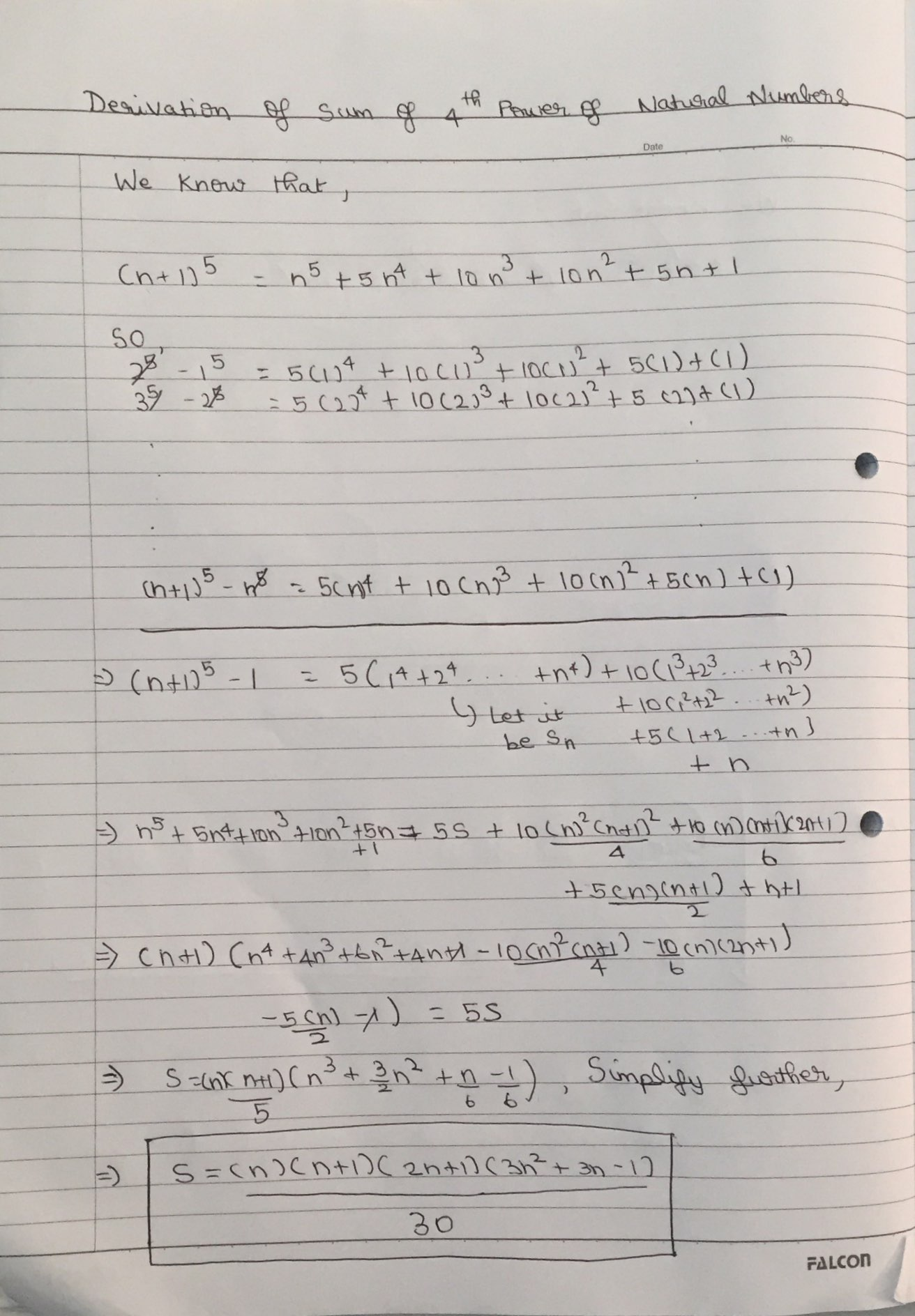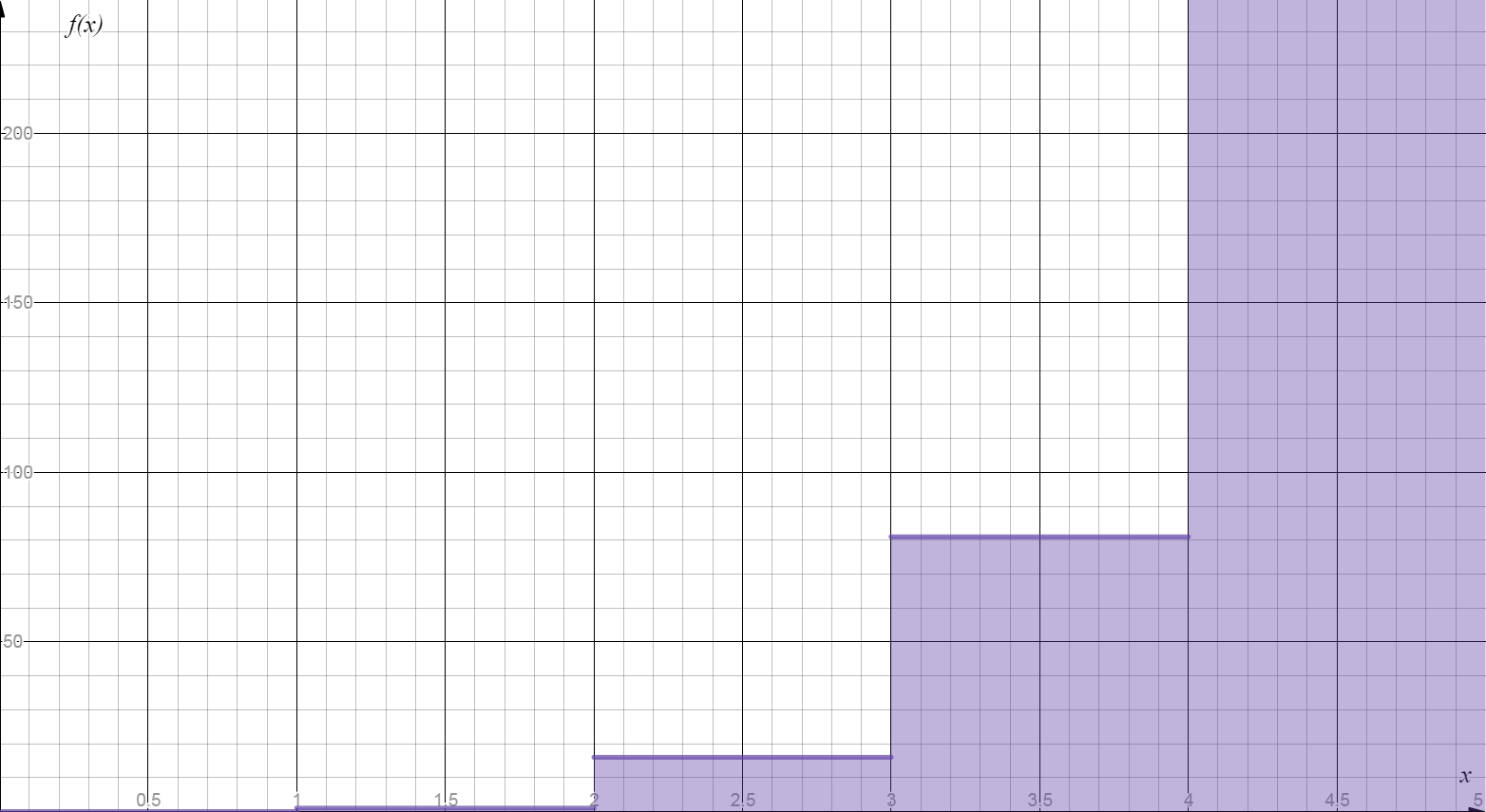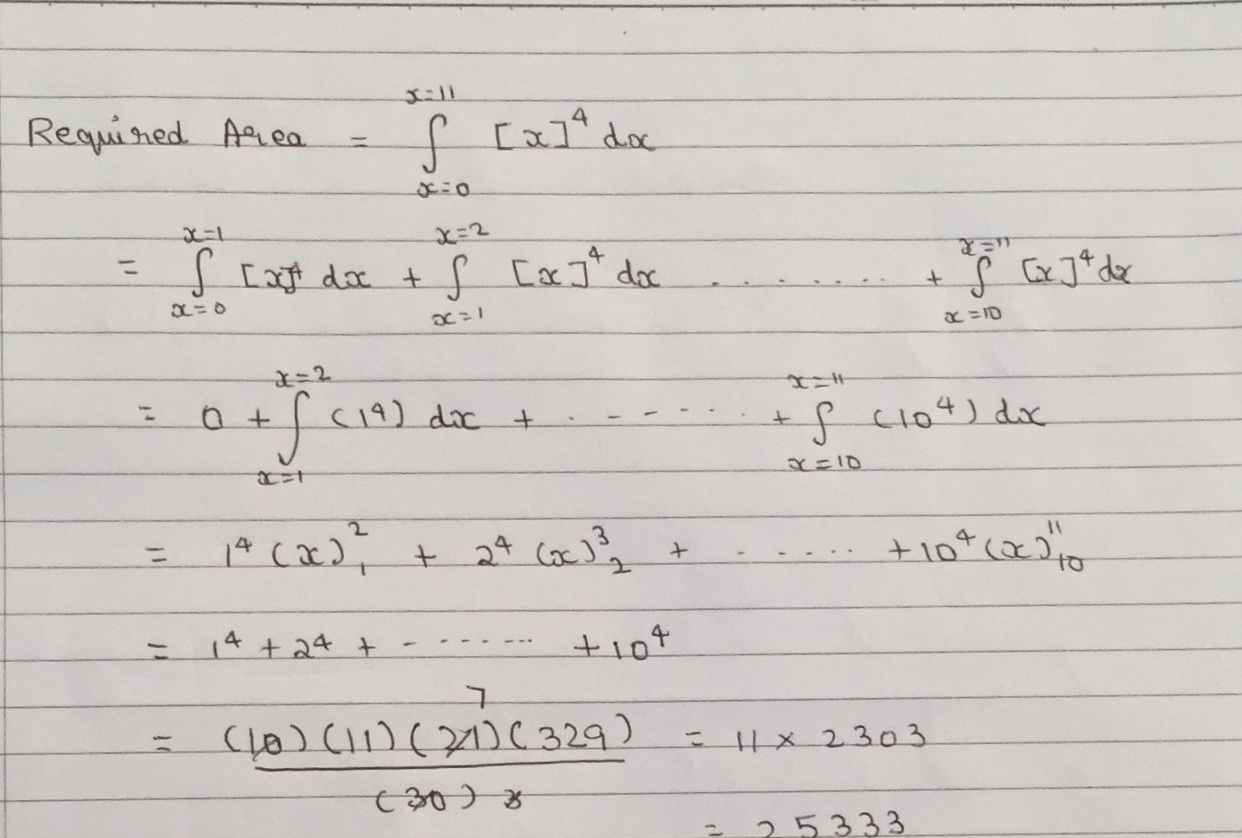# General way to find $\sum _{ x=1 }^{ i }{ { x }^{ n } } ,\quad n\in N$.

Let $\sum _{ x=1 }^{ i }{ { x }^{ n } } ,\quad n\in N=f(n)$.

Using the following fact and a bit of working around we can find f(n).

That is, $(1+x)^\alpha = \sum_{k=0}^{\alpha} \binom{\alpha}{k} x^k$ , where $\binom{\alpha}{k} = \frac{\alpha(\alpha-1)\cdots(\alpha-k+1)}{k!}.$

## For finding f(n) we must know f(1),f(2),......f(n-1).

The following method illustrates the way to find the sum of 4th power of natural numbers , the same can be used for finding for any nth power..

## To try a problem based on this go below.

Find the area bounded between $y = \lfloor x\rfloor ^4$, the $x$-axis, $x = 0$ and $x=11$.##Note by Sanath Balaji
4 years, 5 months ago

This discussion board is a place to discuss our Daily Challenges and the math and science related to those challenges. Explanations are more than just a solution — they should explain the steps and thinking strategies that you used to obtain the solution. Comments should further the discussion of math and science.

When posting on Brilliant:

• Use the emojis to react to an explanation, whether you're congratulating a job well done , or just really confused .
• Ask specific questions about the challenge or the steps in somebody's explanation. Well-posed questions can add a lot to the discussion, but posting "I don't understand!" doesn't help anyone.
• Try to contribute something new to the discussion, whether it is an extension, generalization or other idea related to the challenge.

MarkdownAppears as
*italics* or _italics_ italics
**bold** or __bold__ bold
- bulleted- list
• bulleted
• list
1. numbered2. list
1. numbered
2. list
Note: you must add a full line of space before and after lists for them to show up correctly
paragraph 1paragraph 2

paragraph 1

paragraph 2

[example link](https://brilliant.org)example link
> This is a quote
This is a quote
    # I indented these lines
# 4 spaces, and now they show
# up as a code block.

print "hello world"
# I indented these lines
# 4 spaces, and now they show
# up as a code block.

print "hello world"
MathAppears as
Remember to wrap math in $$ ... $$ or $ ... $ to ensure proper formatting.
2 \times 3 $2 \times 3$
2^{34} $2^{34}$
a_{i-1} $a_{i-1}$
\frac{2}{3} $\frac{2}{3}$
\sqrt{2} $\sqrt{2}$
\sum_{i=1}^3 $\sum_{i=1}^3$
\sin \theta $\sin \theta$
\boxed{123} $\boxed{123}$

Sort by:

There's another method also known as Faulhaber's Formula.

- 3 years, 4 months ago